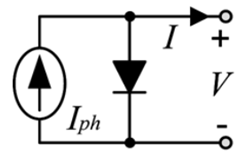# Photovoltaic Cell Model

The photovoltaic (PV) cell converts solar energy into electrical energy (direct current). It is often useful to take a cell operating at a certain solar irradiance and temperature and calculate its electrical output characteristics (i.e. voltage-current (V-I) curve). It is also desirable to perform these calculations using commonly available manufacturer data, e.g. open circuit voltage, short circuit current, voltage and current at maximum power point.

In this article, we will look at several models to approximate the V-I characteristics of a photovoltaic cell.

## Solar Irradiance and Temperature Corrections

The parameters of a PV cell found in manufacturer data sheets are typically quoted at Standard Test Conditions (STC): an irradiance of 1,000 $W/m^{2}$, the standard reference spectral irradiance with Air Mass 1.5 (see the NREL site for more details) and a cell temperature of 25 deg C.

When a PV cell is operating at different values of irradiance and temperature, the manufacturer parameters must first be corrected as follows:

$V_{oc}=V_{oc,STC}\times {\frac {\ln {E}}{\ln {1000}}}\left[1+\alpha (\theta -25)\right]\,$$I_{sc}=I_{sc,STC}\times {\frac {E}{1000}}\left[1+\beta (\theta -25)\right]\,$$V_{mpp}=V_{mpp,STC}\times {\frac {\ln {E}}{\ln {1000}}}\left[1+\alpha (\theta -25)\right]\,$$I_{mpp}=I_{mpp,STC}\times {\frac {E}{1000}}\left[1+\beta (\theta -25)\right]\,$Where $V_{oc}\,$is the cell open circuit voltage (Vdc)

$I_{sc}\,$is the cell short circuit current (A)
$V_{mpp}\,$is the cell voltage at maximum power point (Vdc)
$I_{mpp}\,$is the cell current at maximum power point (A)
$X_{STC}\,$refers to the parameter X at standard test conditions, e.g. $V_{oc,STC}\,$is the open circuit voltage at STC
$E\,$is the actual operating irradiance ($W/m^{2}$)
$\theta \,$is the actual operating temperature (deg C)
$\alpha \,$is the temperature coefficient for voltage (pu)
$\beta \,$is the temperature coefficient for current (pu)

## Approximation of PV Cell V-I Characteristics

The single diode model (SDM) with the equivalent circuit as shown in the figure right is a simple model that is commonly used because of its practicality and the fact that it represents a reasonable compromise between accuracy and simplicity . For a given voltage (V), the current from the cell (I) is approximated by the following well-known equation:

$I=I_{ph}-I_{s}\left(\exp \left[{\frac {V+R_{s}I}{V_{T}}}\right]-1\right)-{\frac {V+R_{s}I}{R_{sh}}}\,$(1)

Where $I_{ph}\,$is the photon current

$I_{s}\,$is the reverse saturation current
$V_{T}\,$is the thermal voltage
$R_{s}\,$is the series resistance
$R_{sh}\,$is the shunt resistance

Notice that this equation does not include the parameters commonly provided by a PV cell / module manufacturer (e.g. open circuit voltage, short circuit current, etc), which makes it limited in terms of practical value. A number of approaches have been proposed in the literature to convert this equation into a form such that a PV cell can be modelled (i.e. derive the V-I characteristics) using only the manufacturer data. The different approaches are typically approximations of the SDM with simplifying assumptions made to make the final equations somewhat tractable. In the subsections below, several of these approaches are presented.

### ISDM ApproximationFigure 2. Ideal Single Diode Model (ISDM)

The ideal single diode model (ISDM) shown in the figure right is a simplification of the SDM, neglecting the influence of the series and shunt losses $R_{s}\,$and $R_{sh}\,$. The ISDM is the simplest model and is not highly accurate, particularly because the series resistance is neglected.

Key Assumptions of the ISDM Approximation:

• Shunt resistance $R_{sh}\,$is infinite at operating points near MPP
• Series resistance $R_{s}\,$is zero
• $\exp \left[{\frac {V+R_{s}I}{V_{T}}}\right]-1>>1$for all operating points
• The photon current is equal to the short circuit current, i.e. $I_{ph}=I_{sc}\,$If we apply these assumptions to Equation (1) above, we get the following reduced equation:

$I=I_{sc}-I_{s}\exp \left[{\frac {V}{V_{T}}}\right]\,$(2)

At open circuit, the current is equal to zero. Therefore:

$I_{sc}=I_{s}\exp \left[{\frac {V_{oc}}{V_{T}}}\right]\,$Re-arranging, we can solve for $I_{s}\,$:

$I_{s}=I_{sc}\exp \left[-{\frac {V_{oc}}{V_{T}}}\right]\,$Plugging the above equation into Equation (2), we get:

$I=I_{sc}\left(1-\exp \left[{\frac {V-V_{oc}}{V_{T}}}\right]\right)\,$(3)

At maximum power point (MPP), the cell voltage and current is known from the manufacturer data sheet as $V_{mpp}\,$and $I_{mpp}\,$respectively. Putting these values into Equation (3):

$I_{mpp}=I_{sc}\left(1-\exp \left[{\frac {V_{mpp}-V_{oc}}{V_{T}}}\right]\right)\,$Re-arranging, we can solve for $V_{T}\,$:

$V_{T}={\frac {V_{mpp}-V_{oc}}{\ln {\left(1-{\frac {I_{mpp}}{I_{sc}}}\right)}}}\,$:

Finally, we get the V-I characteristic of the PV cell by plugging the above expression into Equation (3):

$I=I_{sc}\left(1-\exp \left[{\frac {\ln {\left(1-{\frac {I_{mpp}}{I_{sc}}}\right)}(V-V_{oc})}{V_{mpp}-V_{oc}}}\right]\right)\,$